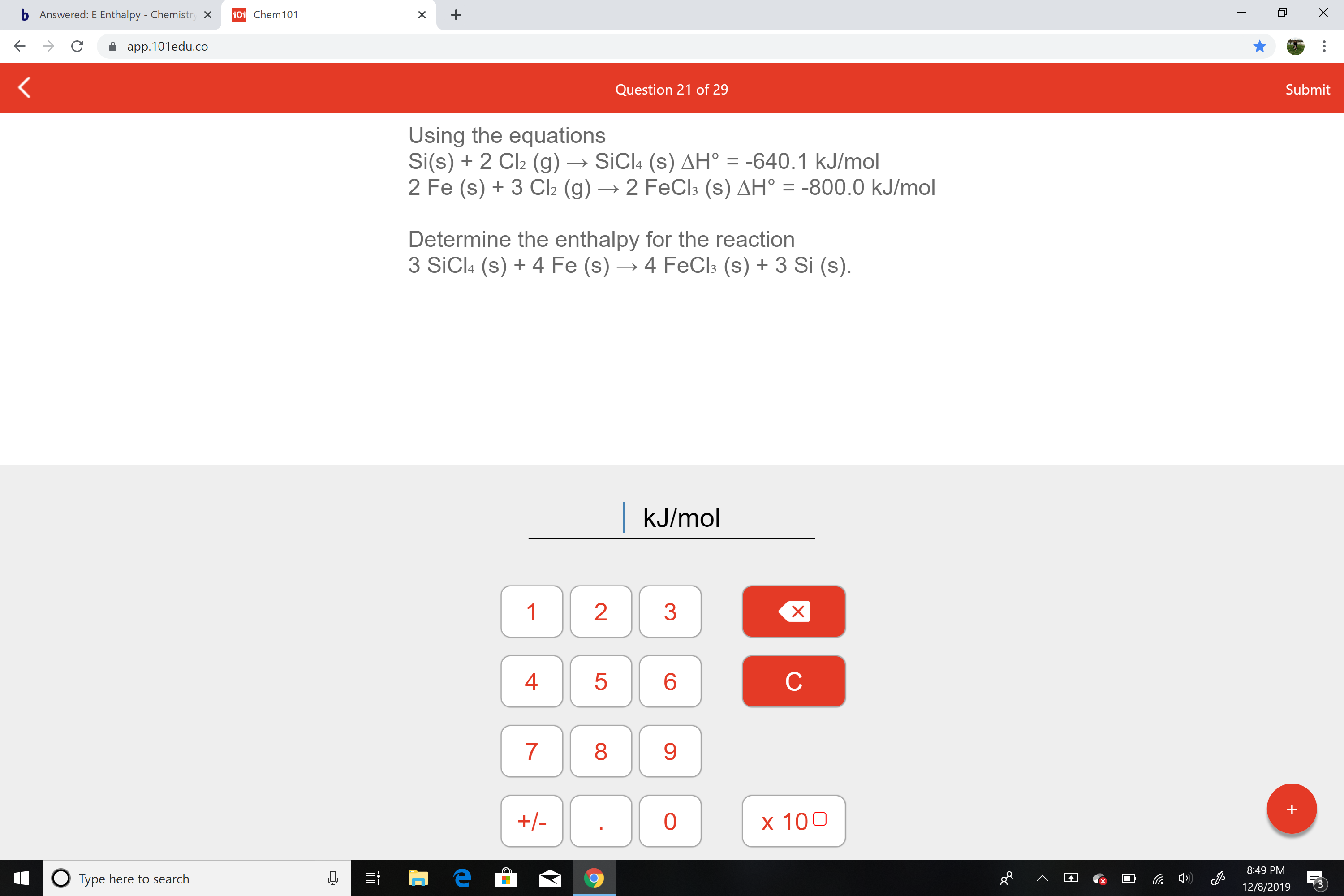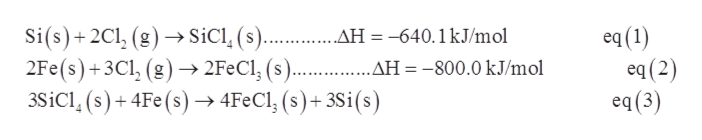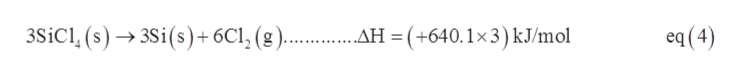# b Answered:E Enthalpy - Chemistry x101 Chem101app.101edu.coSubmitQuestion 21 of 29Using the equationsSi(s) + 2 Cl2 (g) → SICI4 (s) AH° = -640.1 kJ/mol2 Fe (s) + 3 Cl2 (g) → 2 FeCl3 (s) AH° = -800.0 kJ/molDetermine the enthalpy for the reaction3 SiCl: (s) + 4 Fe (s) → 4 FECI3 (s) + 3 Si (s).kJ/mol13489.x 100+/-8:49 PMO Type here to search12/8/2019LO

Question
13 viewshelp_outlineImage Transcriptioncloseb Answered:E Enthalpy - Chemistry x 101 Chem101 app.101edu.co Submit Question 21 of 29 Using the equations Si(s) + 2 Cl2 (g) → SICI4 (s) AH° = -640.1 kJ/mol 2 Fe (s) + 3 Cl2 (g) → 2 FeCl3 (s) AH° = -800.0 kJ/mol Determine the enthalpy for the reaction 3 SiCl: (s) + 4 Fe (s) → 4 FECI3 (s) + 3 Si (s). kJ/mol 1 3 4 8 9. x 100 +/- 8:49 PM O Type here to search 12/8/2019 LO fullscreen
check_circle

Step 1

Given information:help_outlineImage TranscriptioncloseSi(s)+ 2Cl, (g) –→ SiCl, (s).. .AH = -640.1kJ/mol 2Fe(s)+3Cl, (g)→ 2F€C1, (s).... .AH = -800.0 kJ/mol 3SİC1, (s) + 4Fe(s) → 4F¢C1; (s)+ 3Si(s) eq (1) eq (2) eq(3) %3D fullscreen
Step 2

In a chemical equation, if the equation is reversed then the sign for enthalpy change for that equation will also changes also if the equation is multiplied by some numerical value then the value for enthalpy change will also get multiplied.

For calculating enthalpy change for equation (3), first equation (1) is reversed and multiplied by 3 as follows:help_outlineImage Transcriptionclose3SiCl, (s) → 3Si(s)+ 6Cl, (g ). . AH = (+640.1×3) kJ/mol eq (4) fullscreen
Step 3

Now multiplying equation (2) by 2 as the number of FeCl3 molecules in target equat...

### Want to see the full answer?

See Solution

#### Want to see this answer and more?

Solutions are written by subject experts who are available 24/7. Questions are typically answered within 1 hour.*

See Solution
*Response times may vary by subject and question.
Tagged in
ScienceChemistry

### Physical Chemistry